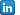February 2019

I recently came across a problem of testing if the expectations of one variable, call it \$Y\$, vary alongside the distribution of another variable, say \$X\$. The problem can be approached through several angles, including parametric quantile approach, however, it was decided to use one of the most flexible methods, and actually one of my favorites, i.e. the bootstrap.

The idea is quite simple. Imagine two random variables \$Y\$ and \$X\$. (For more information about the exact definitions of what a random variable is, the Wikipedia page has a lot of useful information.) Given their observed realisations \$\{(Y_i,X_i):i=1,...,n\}\$, the goal is to test if the conditional average of \$Y\$ is statistically different from its unconditional average. We can approximate the former by estimating the mean of \$Y\$ for different parts of \$X\$ distribution. For instance, we can test if expectations of \$Y\$ d...

Pages: [ 1 ]Marcin Wolski, PhD
Economist
European Investment Bank
E-mail: M.Wolski (at) eib.org
Phone: +352 43 79 88708View my profileIDEAS/RePEc

Categories